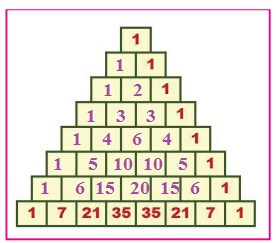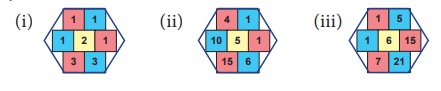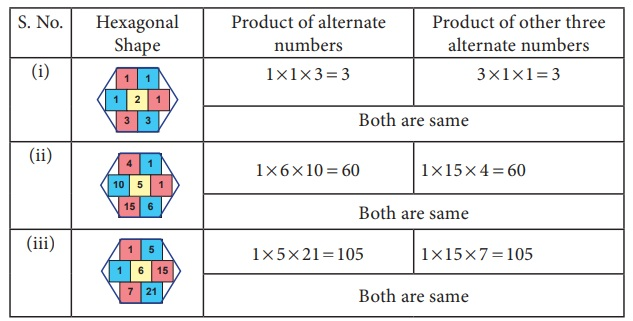Home | | Maths 7th Std | PascalŌĆÖs Triangle

# PascalŌĆÖs Triangle

The triangle of numbers created by the famous French Mathematician and philosopher Blaise Pascal which is named after him as PascalŌĆÖs Triangle.

PascalŌĆÖs Triangle

The triangle of numbers created by the famous French Mathematician and philosopher Blaise Pascal which is named after him as PascalŌĆÖs Triangle. This PascalŌĆÖs Triangle of numbers provides lot of scope to observe various types of number patterns in it.

Activity

1. Complete the following PascalŌĆÖs Triangle by observing the number pattern.Solution:2. Observe the above completed PascalŌĆÖs Triangle and moving the slanting strips, find the sequence that you see in it and complete them. One is done for you(i) 1, 2, 3, 4, 5, 6, 7.

(ii) 1, 3, 6, 10, 15, 21.

(iii) 1, 4, 10, 20, 35.

(iv) 1, 5, 15, 35.

3. Observe the sequence of numbers obtained in the 3rd and 4th slanting rows of PascalŌĆÖs Triangle and find the difference between the consecutive numbers and complete the table given below.Solution:Example 5.2

Tabulate the 3rd slanting row of the PascalŌĆÖs Triangle by taking the position of the numbers in the slanting row as x and the corresponding values as y.Verify whether the relationship, y = [ x(x+1) ] /2 between x and y for the given values is true.

Solution

Observe the table carefully. To verify the relationship between x and y, let us substitute the values of x and get the values of y.Think

The values of y are obtained by half of the product of the two consecutive values of x.

Example 5.3

Can row sum of elements in a PascalŌĆÖs Triangle form a pattern?

Solution

The row sum of elements of a PascalŌĆÖs Triangle are shown below:First row = 21ŌłÆ1 =1

Second row = 22-1=2 ├Ś 1=2

Third row =23-1=2 ├Ś 2=4

Fourth row ==24-1=2 ├Ś 2 ├Ś 2=8

Fifth row = 25-1=2 ├Ś 2 ├Ś 2 ├Ś 2=16

Sixth row = 26-1=2 ├Ś 2 ├Ś 2 ├Ś 2 ├Ś 2=32

Seventh row = 27-1=2 ├Ś 2 ├Ś 2 ├Ś 2 ├Ś 2 ├Ś 2=64

Eighth row = 28-1=2 ├Ś 2 ├Ś 2 ├Ś 2 ├Ś 2 ├Ś 2 ├Ś 2=128

Here x denotes the row and y denotes the corresponding row sum. The values of x and y can be tabulated as follows:The relationship between x and y is y = 2xŌĆō1.

DO YOU KNOW

Observe the pattern obtained by adding the elements in the slanting rows of the PascalŌĆÖs Triangle.The sequence obtained is known as Fibonacci sequence.

Try these

1. Observe the pattern of numbers given in the slanting rows earlier and complete the PascalŌĆÖs Triangle.Solution :2. Complete the given PascalŌĆÖs Triangle. Find the common property of the numbers filled by you. Can you relate this pattern with the pattern discussed in situation 2. Discuss.Solution :Example 5.4

Observe the numbers in the hexagonal shape given in the PascalŌĆÖs Triangle. The product of the alternate three numbers in the hexagon is equal to the product of remaining three numbers. Verify thisSolutionThink

The numbers 1, 3, 6, 10, ... form triangles and are known as triangular numbers. How?

Tags : Information Processing | Term 2 Chapter 5 | 7th Maths , 7th Maths : Term 2 Unit 5 : Information Processing
Study Material, Lecturing Notes, Assignment, Reference, Wiki description explanation, brief detail
7th Maths : Term 2 Unit 5 : Information Processing : PascalŌĆÖs Triangle | Information Processing | Term 2 Chapter 5 | 7th Maths